# 21 1 CREATING AND MEASURING ELECTRIC FIELDS Electric

• Slides: 1021 -1 CREATING AND MEASURING ELECTRIC FIELDS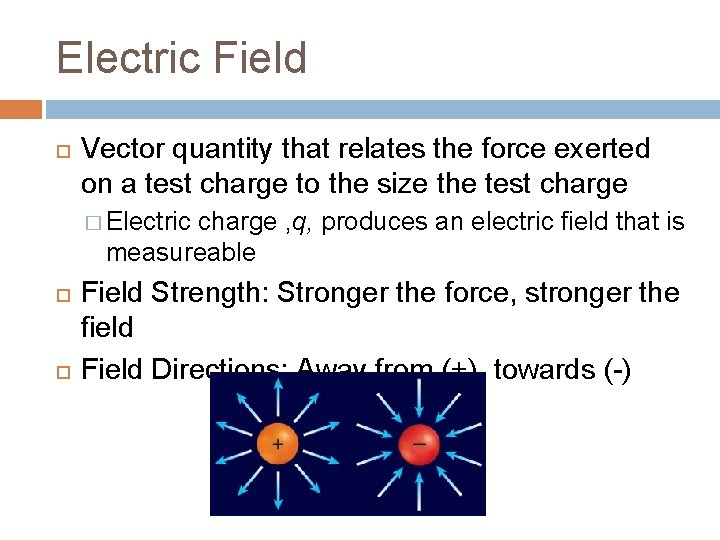Electric Field Vector quantity that relates the force exerted on a test charge to the size the test charge � Electric charge , q, produces an electric field that is measureable Field Strength: Stronger the force, stronger the field Field Directions: Away from (+), towards (-)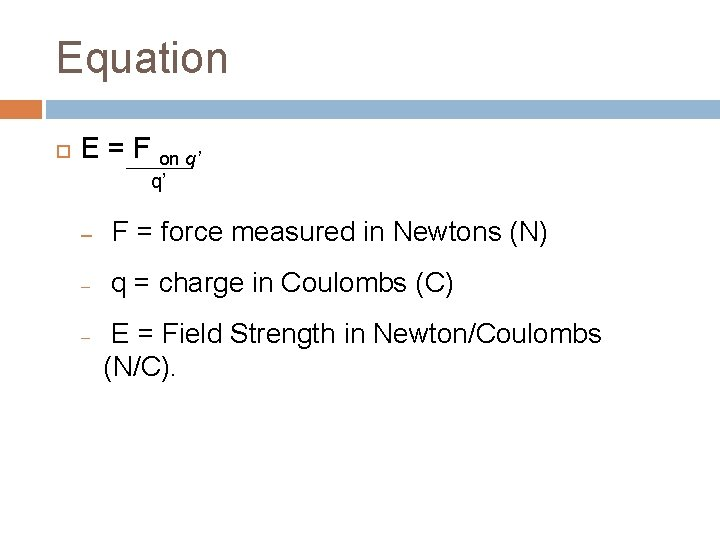Equation E = F on q’ q’ – F = force measured in Newtons (N) – q = charge in Coulombs (C) – E = Field Strength in Newton/Coulombs (N/C).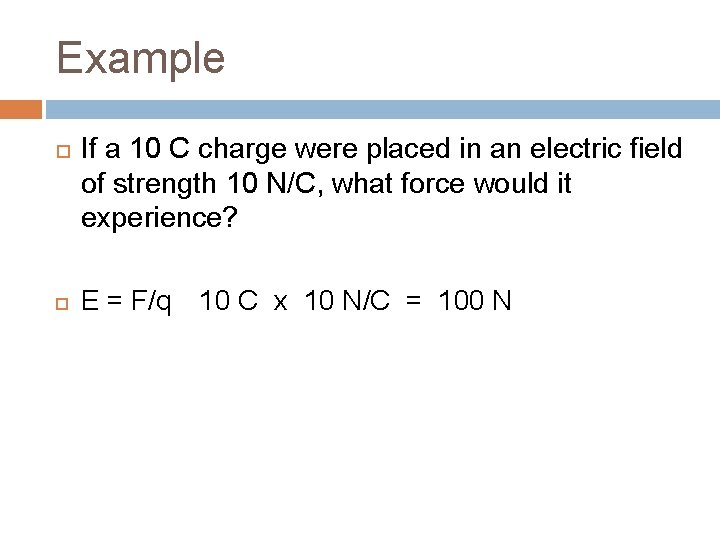Example If a 10 C charge were placed in an electric field of strength 10 N/C, what force would it experience? E = F/q 10 C x 10 N/C = 100 NExample An electric field is to be measured using a positive test charge of 4. 0 x 10 -5 C. This test charge experiences a force of 0. 60 N acting at an angle of 10 o. What is the magnitude and direction of the electric field at the location of the test charge? Known: Unknown �q = +4. 0 x 10 -5 C � F = 0. 60 N at 10 o E = ? ? ? At 10 oE=F/q 0. 60 N / 4. 0 x 10 -5 C E = 1. 5 x 104 N/C at 10 o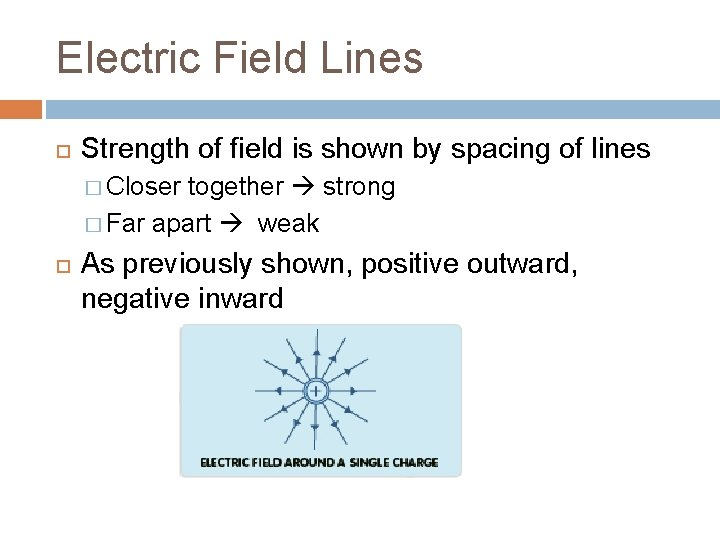Electric Field Lines Strength of field is shown by spacing of lines � Closer together strong � Far apart weak As previously shown, positive outward, negative inward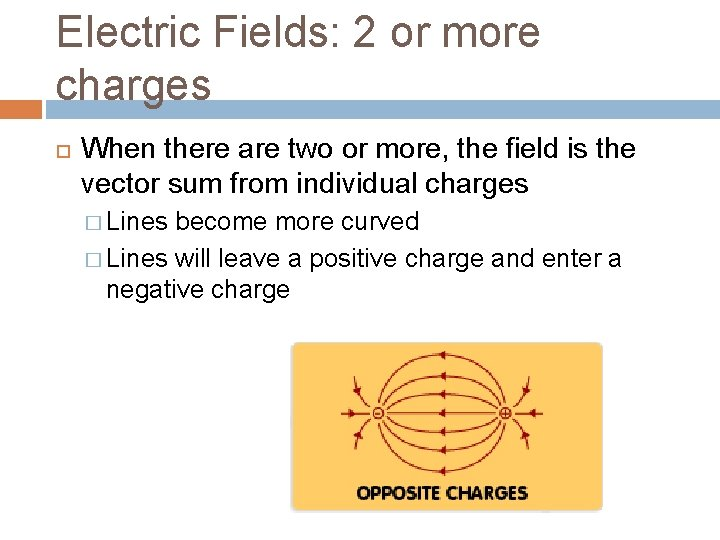Electric Fields: 2 or more charges When there are two or more, the field is the vector sum from individual charges � Lines become more curved � Lines will leave a positive charge and enter a negative charge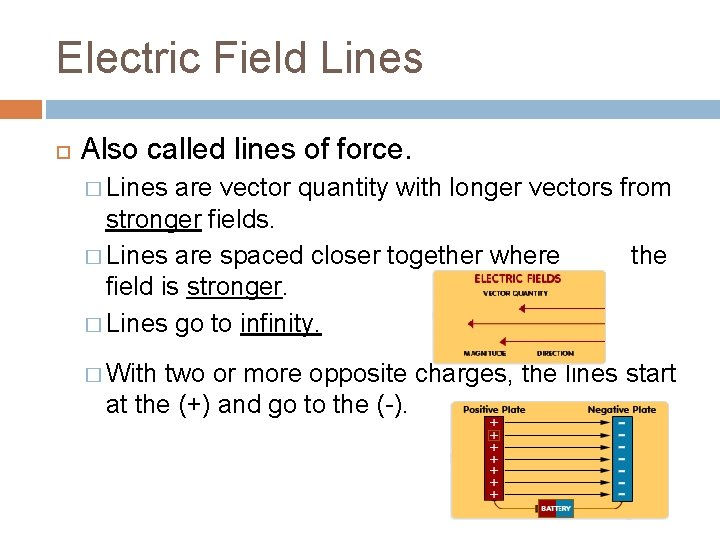Electric Field Lines Also called lines of force. � Lines are vector quantity with longer vectors from stronger fields. � Lines are spaced closer together where the field is stronger. � Lines go to infinity. � With two or more opposite charges, the lines start at the (+) and go to the (-).Van de Graff machine Transfers large amounts of charge from one part of the machine to the top meal terminal � Person touches it becomes charged electrically and the charges repel *stands hair up*